# Arduino Ultrasonic Distance Sensor Project

Author: Aus Electronics Direct   Date Posted:3 April 2019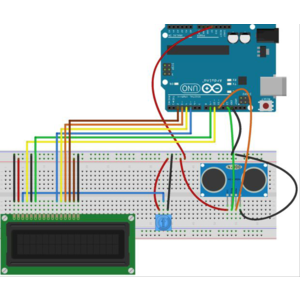# Arduino Ultrasonic Distance Measurement Sensor Project

In this Arduino Tutorial we will learn how to use the HC-SR04 Ultrasonic Sensor to display the distance measured on LCD.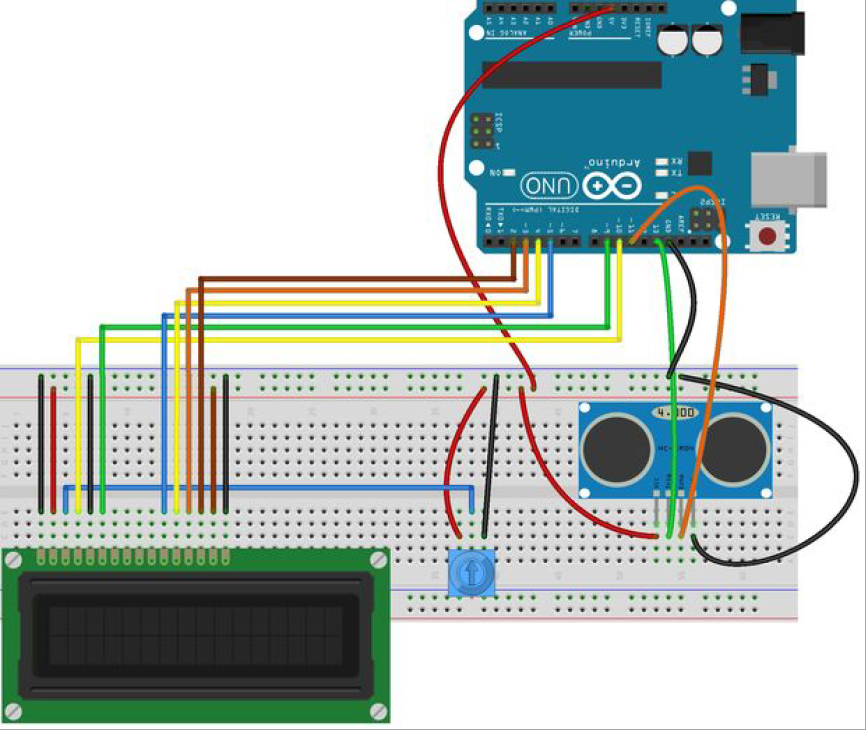The objective of this project is to measure distance using the HC-SR04 Ultrasonic Sensor and display the value on the 2 x 16 LCD module display.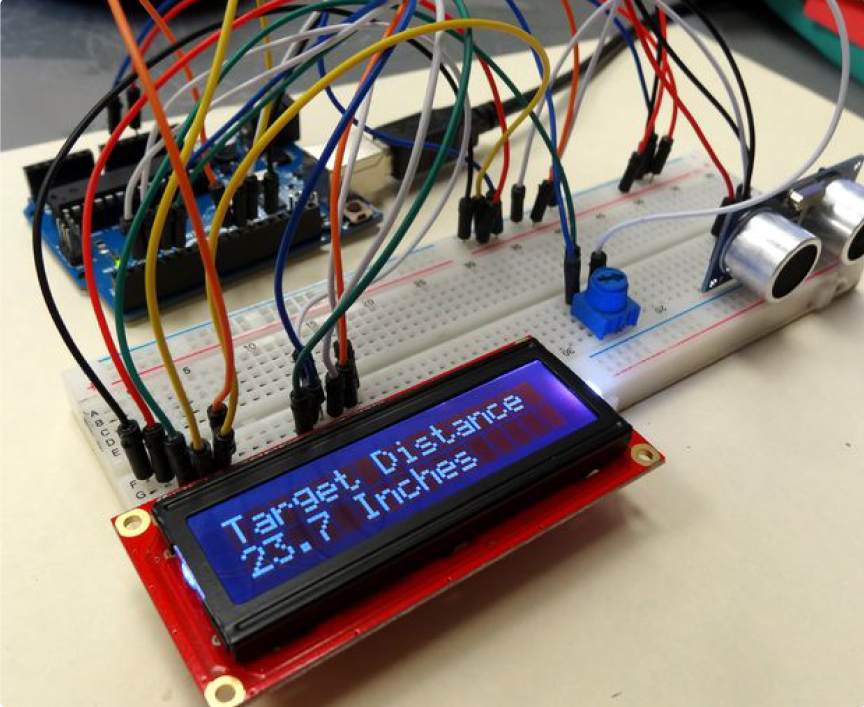Parts Required :

- TA0000 Uno Development board
- TA0037  Ultrasonic Sensor
- 5k  Potentiomenter
- TA0152 2 x 16 LCD Display Module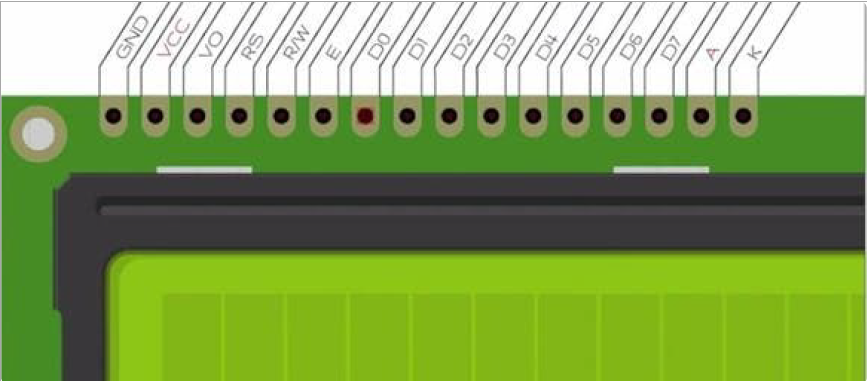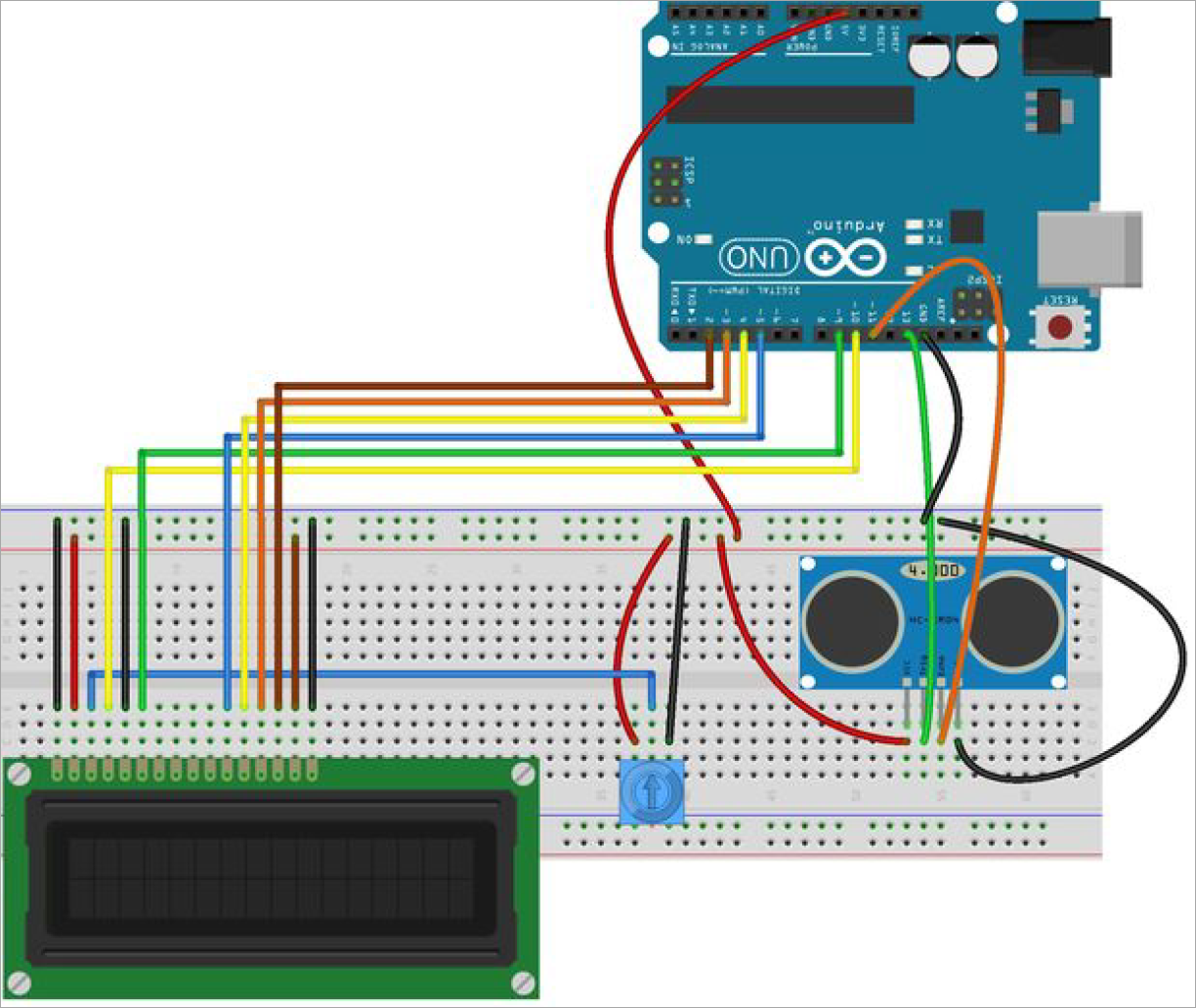Wiring:
- 2 x 16 LCD Module:
Connect the Cathode to Arduino GND
Anode to arduino 5v
D7 to Arduino pin 2
D6 to Arduino pin 3
D5 to Arduino pin 4
D4 to arduino pin 5
E to Arduino pin 9
R/W to Arduino GND
RS to Arduino pin 10
VO to the pot
Vcc to Arduino 5v
GND to Arduino GND
Connecting the Ultrasonic sensor:
Vcc to Arduino 5v
Trigger to Arduino pin 13
Echo to Arduino pin 11
Gnd to Arduino GND
- Connect the potentiometer to GND and Vcc as shown in the wiring diagram above

Arduino Code

#include <LiquidCrystal.h> //Load Liquid Crystal Library

LiquidCrystal LCD(10, 9, 5, 4, 3, 2); //Create Liquid Crystal Object called LCD

int trigPin=13; //Sensor Trip pin connected to Arduino pin 13

int echoPin=11; //Sensor Echo pin connected to Arduino pin 11

int myCounter=0; //declare your variable myCounter and set to 0

int servoControlPin=6; //Servo control line is connected to pin 6

float pingTime; //time for ping to travel from sensor to target and return

float targetDistance; //Distance to Target in inches

float speedOfSound=776.5; //Speed of sound in miles per hour

void setup() {

Serial.begin(9600);

pinMode(trigPin, OUTPUT);

pinMode(echoPin, INPUT);

LCD.begin(16,2); //Tell Arduino to start your 16 column 2 row LCD

LCD.setCursor(0,0); //Set LCD cursor to upper left corner, column 0, row 0

LCD.print("Target Distance:"); //Print Message on First Row

}

void loop() {

digitalWrite(trigPin, LOW); //Set trigger pin low

delayMicroseconds(2000); //Let signal settle

digitalWrite(trigPin, HIGH); //Set trigPin high

delayMicroseconds(15); //Delay in high state

digitalWrite(trigPin, LOW); //ping has now been sent

delayMicroseconds(10); //Delay in high state

pingTime = pulseIn(echoPin, HIGH); //pingTime is presented in microceconds

pingTime=pingTime/1000000; //convert pingTime to seconds by dividing by 1000000 (microseconds in a second)

pingTime=pingTime/3600; //convert pingtime to hourse by dividing by 3600 (seconds in an hour)

targetDistance= speedOfSound * pingTime; //This will be in miles, since speed of sound was miles per hour targetDistance=targetDistance/2; //Remember ping travels to target and back from target.

targetDistance= targetDistance*63360; //Convert miles to inches by multipling by 63360 (inches per mile)

LCD.setCursor(0,1); //Set cursor to first column of second row

LCD.print(" "); //Print blanks to clear the row

LCD.setCursor(0,1); //Set Cursor again to first column of second row

LCD.print(targetDistance); //Print measured distance

delay(250); //pause to let things settle

}